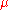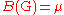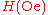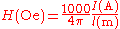xOerstedEncyclopedia
Oersted is the unit of magnetizing field (also known as H-field, magnetic field strength or intensity) in the CGS
Centimetre gram second system of units
The centimetre–gram–second system is a metric system of physical units based on centimetre as the unit of length, gram as a unit of mass, and second as a unit of time...

system of units.

## Difference between cgs and SI systems

In cgs the unit of H-field is the oersted and the unit of B-field is the gauss
Gauss (unit)
The gauss, abbreviated as G, is the cgs unit of measurement of a magnetic field B , named after the German mathematician and physicist Carl Friedrich Gauss. One gauss is defined as one maxwell per square centimeter; it equals 1 tesla...

. In the SI system, Ampere per meter (A/m) is used for H-field and Tesla
Tesla (unit)
The tesla is the SI derived unit of magnetic field B . One tesla is equal to one weber per square meter, and it was defined in 1960 in honour of the inventor, physicist, and electrical engineer Nikola Tesla...

is used for B-field.

## History

The unit was established by the IEC
International Electrotechnical Commission
The International Electrotechnical Commission is a non-profit, non-governmental international standards organization that prepares and publishes International Standards for all electrical, electronic and related technologies – collectively known as "electrotechnology"...

in 1930 in honour of the Danish physicist Hans Christian Ørsted
Hans Christian Ørsted
Hans Christian Ørsted was a Danish physicist and chemist who discovered that electric currents create magnetic fields, an important aspect of electromagnetism...

.

## Definition

The oersted is defined as an dyne
Dyne
In physics, the dyne is a unit of force specified in the centimetre-gram-second system of units, a predecessor of the modern SI. One dyne is equal to exactly 10 µN...

per unit pole
Magnetic pole strength
Magnetic pole strength is a physical quantity used to measure the strength of the pole of a bar magnet...

. The Oersted is 1000/4π (≈79.5774715) ampere per meter, in terms of SI units.

So, the H-field strength inside a long solenoid
Solenoid
A solenoid is a coil wound into a tightly packed helix. In physics, the term solenoid refers to a long, thin loop of wire, often wrapped around a metallic core, which produces a magnetic field when an electric current is passed through it. Solenoids are important because they can create...

wound with 79.58 turns per meter of a wire carrying 1 A
Ampere
The ampere , often shortened to amp, is the SI unit of electric current and is one of the seven SI base units. It is named after André-Marie Ampère , French mathematician and physicist, considered the father of electrodynamics...

will be approximately 1 oersted. The preceding statement is exactly correct if the solenoid considered is infinite in length and the current evenly distributed over its surface.

The oersted is closely related to the gauss
Gauss (unit)
The gauss, abbreviated as G, is the cgs unit of measurement of a magnetic field B , named after the German mathematician and physicist Carl Friedrich Gauss. One gauss is defined as one maxwell per square centimeter; it equals 1 tesla...

, the CGS unit of magnetic flux density. In a vacuum, if the magnetizing field strength is 1 Oe, then the magnetic field density is 1 Gs, whereas in a medium having permeabilityr (relative to permeability of vacuum), their relation is:r *Because oersteds are used to measure magnetizing field strength, they are also related to magnetomotive force
Magnetomotive force
Magnetomotive force is any physical driving force that produces magnetic flux. In this context, the expression "driving force" is used in a general sense of "work potential", and is analogous, but distinct from force measured in newtons...

(mmf) of current in a single-winding wire-loop:The stored energy in a magnet, called magnet performance or maximum energy product (often abbreviated BHmax), is typically measured in units of megagauss-oersteds (MGOe). One MGOe is approximately equal to 7957.74715 J/m3.